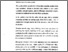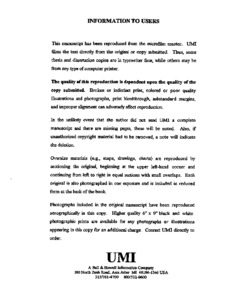Title:

# Construction of the orthogonal groups of n x n circulant matrices over finite fields

Zhang, Zhe (1997) Construction of the orthogonal groups of n x n circulant matrices over finite fields. Masters thesis, Concordia University.Preview
Text (application/pdf)
MQ25987.pdf
2MB

## Abstract

Let F be a finite field with q elements where $q=p\sp{m}, p$ prime. Let ${\cal M}$ be the algebra of n x n circulant matrices over F. The set $O\sb{(n,q)}$ of orthogonal n x n circulant matrices is a subgroup of ${\cal M}\sp\times.$ The major purposes of the thesis are: (1) to explain K. A. Byrd and T. P. Vaughan's results stated in (8), about formulas for the orders, and algorithms for the construction, of the groups $O\sb{(n,q)};$ (2) to show new examples and develop programs to find the orders and to actually construct the group $O\sb{(n,q)}$ for any given n and q.

Divisions: Concordia University > Faculty of Arts and Science > Mathematics and Statistics Thesis (Masters) Zhang, Zhe v, 77, 30 leaves ; 29 cm. Concordia University M.Sc. Mathematics 1997 Francisco, Thaine QA 188 Z47 1997 264 Concordia University Library 27 Aug 2009 17:10 13 Jul 2020 19:46 https://concordiauniversity.on.worldcat....
All items in Spectrum are protected by copyright, with all rights reserved. The use of items is governed by Spectrum's terms of access.

Repository Staff Only: item control pageResearch related to the current document (at the CORE website)
Back to top